# pendulum

(redirected from Huygens law)
Also found in: Dictionary, Thesaurus.
Related to Huygens law: Huygens principle

## pendulum,

a mass, called a bob, suspended from a fixed point so that it can swing in an arc determined by its momentum and the force of gravity. The length of a pendulum is the distance from the point of suspension to the center of gravity of the bob (see center of masscenter of mass,
the point at which all the mass of a body may be considered to be concentrated in analyzing its motion. The center of mass of a sphere of uniform density coincides with the center of the sphere.
). Chance observation of a swinging church lamp led Galileo to find that a pendulum made every swing in the same time, independent of the size of the arc. He used this discovery in measuring time in his astronomical studies. His experiments showed that the longer the pendulum, the longer is the time of its swing. Christiaan HuygensHuygens, Christiaan
, 1629–95, Dutch mathematician and physicist; son of Constantijn Huygens. He improved telescopic lenses and discovered (1655) a satellite of Saturn and studied the rings of Saturn. His findings were described in his Systema Saturnium (1659).
determined an exact relation between the length of the pendulum and the time of vibration when the arc of swing is small. He arrived at the formula T = 2π√l/g, where T is the period, or time for one complete swing, l is the length, g is the acceleration of gravity (see gravitationgravitation,
the attractive force existing between any two particles of matter. The Law of Universal Gravitation

Since the gravitational force is experienced by all matter in the universe, from the largest galaxies down to the smallest particles, it is often called
), and π = 3.14 … (see harmonic motionharmonic motion,
regular vibration in which the acceleration of the vibrating object is directly proportional to the displacement of the object from its equilibrium position but oppositely directed.
). In 1673, Huygens devised a practicable means of making a pendulum control the speed with which a clock mechanism runs. This impetus to clockmaking resulted not only in the development of many types of clock, such as the "wag at the wall," the grandfather clock, and the banjo clock, but also in the application of pendulum control to other mechanisms. Metal pendulums are lengthened by heat; to counteract the effect of temperature changes, compensation pendulums have been devised, many of them operating by the opposite expansion of different metals in compound rods. Forces acting on the bob, such as air resistance, affect its swing. Since gravity varies from place to place, the pendulum has been used to determine the shape and mass of the earth by measuring the intensity of gravity. In the seismograph (see seismologyseismology
, scientific study of earthquakes and related phenomena, including the propagation of waves and shocks on or within the earth by natural or artificially generated seismic signals.
), a pendulum registers the direction of an earthquake. In 1851, J. B. L. FoucaultFoucault, Jean Bernard Léon
, 1819–68, French physicist. Known especially for his research on the speed of light, he determined its velocity in air and found that its speed in water and other media diminished in proportion to the index of refraction.
demonstrated the rotation of the earth by suspending in the Panthéon in Paris a 200-ft (61-m) pendulum that traced its path in sand on the floor. The pendulum continued to vibrate in a single plane as the earth rotated underneath it, thus leaving a series of traces in the sand in all directions. A pendulum made to swing in a circle, describing a cone, is called a conical pendulum. In the torsion pendulum the bob vibrates by twisting and untwisting, as in the balance wheel of a watch.

## Pendulum

A rigid body mounted on a fixed horizontal axis, about which it is free to rotate under the influence of gravity. The period of the motion of a pendulum is virtually independent of its amplitude and depends primarily on the geometry of the pendulum and on the local value of g, the acceleration of gravity. Pendulums have therefore been used as the control elements in clocks, or inversely as instruments to measure g.

#### Motion

In the schematic representation of a pendulum shown in the illustration, O represents the axis and C the center of mass. The line OC makes an instantaneous angle Θ with the vertical. In rotary motion of any rigid body about a fixed axis, the angular acceleration is equal to the torque about the axis divided by the moment of inertia I about the axis. If m represents the mass of the pendulum, the force of gravity can be considered as the weight mg acting at the center of mass C.

If the amplitude of motion is small, the motion is simple harmonic. The period T, time for a complete vibration (for example, from the extreme displacement right to the next extreme displacement right), is given by Eq. (1). See Harmonic motion (1)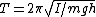The actual form of a pendulum often consists of a long, light bar or a cord that serves as a support for a small, massive bob. The idealization of this form into a point mass on the end of a weightless rod of length L is known as a simple pendulum. An actual pendulum is sometimes called a physical or compound pendulum. In a simple pendulum the lengths h and L become identical, and the moment of inertia I equals mL2. Equation (1) for the period becomes Eq. (2). (2)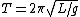#### Center of oscillation

Equation (2) can be used to define the equivalent length of a physcal pendulum. Comparison with Eq. (1) shows that Eq.

(3)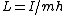(3) holds. The point P on line OC of the illustration, whose distance from the axis O equals L, is called the center of oscillation. Points O and P are reciprocally related to each other in the sense that if the pendulum were suspended at P, O would be the center of oscillation.

#### Types

Kater's reversible pendulum is designed to measure g, the acceleration of gravity. It consists of a body with two knife-edge supports on opposite sides of the center of mass as at O and P (and with at least one adjustable knife-edge). If the pendulum has the same period when suspended from either knife-edge, then each is located at the center of oscillation of the other, and the distance between them must be L, the length of the equivalent simple pendulum. The value for g follows from Eq. (2).

The ballistic pendulum is a device to measure the momentum of a bullet. The pendulum bob is a block of wood into which the bullet is fired. The bullet is stopped within the block and its momentum transferred to the pendulum. This momentum is determined from the amplitude of the pendulum swing. See Ballistics

The spherical pendulum is a simple pendulum mounted on a pivot so that its motion is not confined to a plane. The bob then moves over a spherical surface. A Foucault pendulum is a spherical pendulum suspended so that its plane of oscillation is free to rotate. Its purpose is to demonstrate the rotation of the Earth. See Foucault pendulum

McGraw-Hill Concise Encyclopedia of Physics. © 2002 by The McGraw-Hill Companies, Inc.

## Pendulum

(religion, spiritualism, and occult)

Apendulum is an instrument used in dowsing or radiesthesia. It is basically a weight on the end of a length of cord or fine chain. Radiesthesia may be defined as divination utilizing human sensitivity to radiations emanating from any source, living or inert. It is a refinement of the art of rhabdomancy, or water witching, and its history can be traced back more than 5,000 years to the ancient Orient. The principle instrument used is the pendulum, though rods of various types may also be used. The practice came to the fore during the Middle Ages and remained popular through to the early nineteenth century. It then came to be regarded as a superstition without value, though radiesthesia saw a great resurgence of interest in the twentieth century. The term radiesthesia was coined in France (as radiesthésie) by the Abbé Bouly, in 1930. That same year L Association des Amis de la Radiesthésie was founded and three years later, in England, the British Society of Dowsers.

A radiesthetist is a dowser sensitive to hidden information who uses an indicator, such as a pendulum or rod, to amplify that sensitivity. The pendulum is a weight of some sort suspended on a fine chain or length of thread. Some radiesthetists hold a short stick with the pendulum hanging off the end of it, but most modern day diviners hold the end of the chain directly between their fingers. Virtually any small weight will work; some use a pendant necklace or a ring on the end of a length of silk ribbon. In the Middle Ages a key on the end of a chain was popular. Today there are commercially produced pendula made out of wood, brass, silver, gold, and plastic. A serviceable one can be fashioned from a fishing weight or plumb bob. Some of the commercially produced ones are hollow, and unscrew to give access to an inside cavity. In this can be placed what is known as a “witness;” a sample of what is being sought. For example, if the radiesthetist is looking for gold, then a small piece of gold would be placed inside the pendulum, to help make the connection. The pendulum is often used to diagnose and prescribe for disease. It can also be used to answer questions, in the manner of a talking board.

Most radiesthesia is done seated at a table, working with charts, maps, or other simple accessories. The end of the chain, or thread, is held between thumb and first finger—right hand if right handed; left hand if left-handed—allowing the pendulum weight to be suspended about an inch off the surface of the table. A square of paper, placed under the pendulum, is marked with a large cross and the words YES and NO. Resting the elbow on the table, the weight of the pendulum hangs over the center of the cross and questions are asked that can be answered Yes or No. Although held steadily, the pendulum starts to swing along one of the lines of the cross, giving either a Yes or No answer. An alternate method that does not employ any card or paper is simply for the pendulum to be allowed to swing in a clockwise circle for Yes and counterclockwise for No.

The pendulum should swing of its own accord, much as the planchette of a Ouija® board moves about without being directed. Although it is not consciously made to swing, it is almost certainly the operator’s muscles which are bringing it about. But as with the talking board, the question is, who or what is directing it to give the answers received? To get more than Yes and No answers, the letters of the alphabet may be placed in a semicircle on a table. With the weight of the pendulum hanging in the center, questions can be asked and answers obtained by observing to which letters the pendulum swings. A variation is to hold the pendulum so that it hangs down in the center of a water glass. It can then swing and “chink” against the side of the glass; one hit for A, two for B, three for C, and so on. Again, similar to the message reception obtained from table tipping.

A Swedish medium, Anna Rasmussen—whose powers first became obvious when she was twelve years of age—has affected pendulums by psychokinesis. Professor Winther of Copenhagen studied her intensively between 1922 and 1928. He designed a sealed glass case inside which hung a variety of pendulums. They were of different weights and supported on silk threads. Anna could concentrate on them and cause any one of them, as chosen by the professor, to swing in any direction requested. Anna had a spirit guide named Dr. Lasaruz, whom she claimed brought about the movements.

Sources:

Buckland, Raymond: Color Magic—Unleash Your Inner Powers. St. Paul: Llewellyn, 2002
Holroyd, Stuart: The Supernatural: Minds Without Boundaries. London: Aldus, 1975
Lakhovsky, Georges (tr. Mark Clement): The Secret of Life: Cosmic Rays and Radiations of Living Beings. London: True Health, 1963
Maury, Marguerite: How to Dowse, Experimental and Practical Radiesthesia. London: Bell, 1953
Mermet, Abbé: Principles and Practice of Radiesthesia. London: Watkins, 1975
Wethered, Vernon D.: The Practice of Medical Radiesthesia. London: C.W. Daniel, 1967
The following article is from The Great Soviet Encyclopedia (1979). It might be outdated or ideologically biased.

## Pendulum

a rigid body that under the action of applied forces oscillates about a fixed point or axis. In physics the term “pendulum” usually means a pendulum that oscillates under the action of the force of gravity, with its axis not passing through the body’s cenr of gravity. The simplest pendulum consists of a small, massive bob C suspended on a string or light rod of length l. If the string is assumed to be inextensible and if the dimensions of the bob compared with the length of the string and the mass of the string compared with the mass of the bob are disregarded, then the bob on the string may be considered to be a point mass located at a constant distance l from the point of suspension O (Figure l,a). Such a pendulum is called a simple pendulum. If, however, as is usually the case, the oscillating body cannot be considered to be a point mass, then the pendulum is said to be a compound, or physical, pendulum.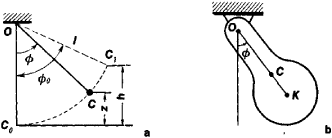Figure 1. Pendulums: (a) simple and (b) compound

Simple pendulum. If a pendulum deflected from its equilibrium position Co is released with zero initial velocity or if a velocity directed perpendicular to OC and lying in the plane of the initial deflection is imparted to the point mass C, then the pendulum will oscillate in a single vertical plane along an arc of a circle (a planar, or circular, simple pendulum). In this case, the position of the pendulum is specified by a single coordinate, for example, the angle cf> through which the pendulum is deflected from the equilibrium position. In the general case, the oscillations of a pendulum are not harmonic; their period T depends on the amplitude. But if the deflections of a pendulum are small, then the pendulum undergoes oscillations that are close to harmonic and have a period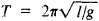where g is the acceleration due to gravity; in this case, the period T is independent of the amplitude, that is, the oscillations are isochronous.

If an initial velocity that does not lie in the plane of the initial deflection is imparted to a pendulum that has been deflected, then on a sphere of radius l the point C will describe curves that lie between the two parallels z = z1 and z = z2 (Figure 2,a), where the values of z1 and Z2 depend on the initial conditions (a spherical pendulum). In the particular case when z1 = z2 (Figure 2,b), the point C will describe a circle in a horizontal plane (a conical pendulum). Of the noncircular pendulums, of particular interest is the cycloidal pendulum, whose oscillations are isochronous for any amplitude.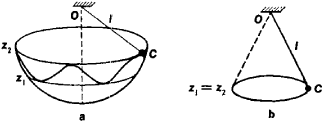Figure 2. Pendulums: (a) spherical and (b) conical

Compound pendulum. A rigid body that under the force of gravity oscillates about a horizontal axis of suspension is usually called a compound pendulum (Figure l,b). The motion of such a pendulum is entirely analogous to the motion of a circular simple pendulum. At small angles of deflection (f>, a compound pendulum undergoes oscillations that are close to harmonic and have a period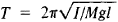where I is the moment of inertia of the pendulum with respect to the axis of suspension, l is the distance from the axis of suspension, O to the cenr of gravity C, and M is the mass of the pendulum. Consequently, the period of oscillation of a compound pendulum coincides with the period of oscillation of a simple pendulum that has the length l0 = I/Ml This length is called the equivalent length of the given compound pendulum.

The point K that lies on the extension of the line OC at a distance l0 from the axis of suspension is called the center of oscillation of the compound pendulum. Here the distance OK = l0 is always greater than OC = l. The point O on the axis of suspension of the pendulum and the center of oscillation K have the property of reciprocity: if the axis of suspension is made to pass through the center of oscillation, then the point O of the former axis of suspension will become the new center of oscillation, and the period of oscillation of the pendulum will not change. This property of reciprocity is used in a reversible pendulum to determine the equivalent length l0; if l,sub>0 and T are known, we can find the value of g at a given point.

The properties of pendulums are widely used in various devices, including clocks; apparatus used to determine the acceleration due to gravity (see), the acceleration of moving bodies, and the oscillations of the earth’s crust; gyroscopic devices; and instruments used to determine experimentally the moments of inertia of bodies.

### REFERENCES

Bukhgol’ts, N. N. Osnovnoi kurs teoreticheskoi mekhaniki. Part 1, Moscow, 1967, sec. 38, pars. 5, 13, and 14. Part 2, Moscow, 1969, sec. 12, par. 4.
Targ, S. M. Kratkii kurs teoreticheskoi mekhaniki, 7th ed. Moscow, 1970. Chapter 28, sec. 155.
Khaikin, S. E. Fizicheskie osnovy mekhaniki, 2nd ed. Moscow, 1971. Chapter 13, sees. 90 and 91.

S. M. TARG

## pendulum

[′pen·jə·ləm]
(physics)
A rigid body mounted on a fixed horizontal axis, about which it is free to rotate under the influence of gravity. Also known as compound pendulum; gravity pendulum.
McGraw-Hill Dictionary of Scientific & Technical Terms, 6E, Copyright © 2003 by The McGraw-Hill Companies, Inc.

## pendulum

a body mounted so that it can swing freely under the influence of gravity. It is either a bob hung on a light thread (simple pendulum) or a more complex structure (compound pendulum)
Collins Discovery Encyclopedia, 1st edition © HarperCollins Publishers 2005
Site: Follow: Share:
Open / Close